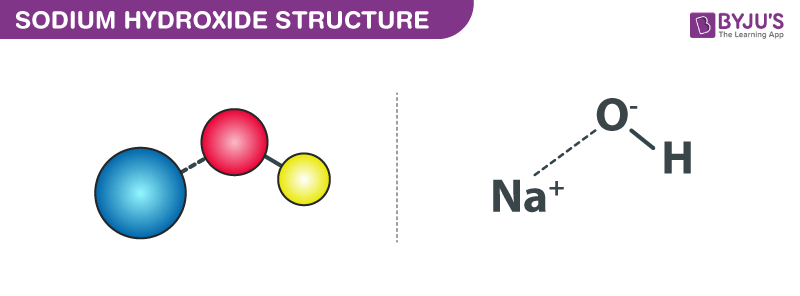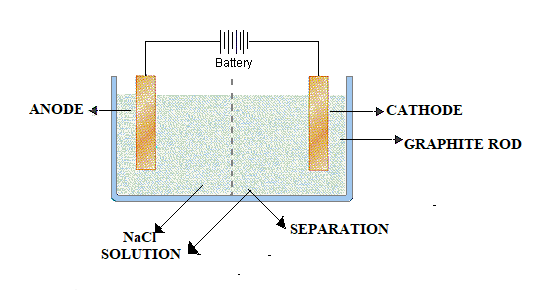# Sodium Hydroxide

Sodium Hydroxide belongs to the category of inorganic compounds and is usually found in the form of a white solid at room temperature. This chemical compound consists of sodium Nacations and hydroxide OH− anions. Sodium Hydroxide chemical formula is written as NaOH. It is also commonly known as caustic soda or Iye and is widely used in manufacturing a variety of products such as paper, soap and detergents, pulp, explosives, liquid drain and oven cleaners, etc.

Sodium Hydroxide is one of the simplest hydroxides. Due to such characteristics, it is often used with neutral water and (acidic) HCl acid to determine the pH scale. We will learn more about Sodium Hydroxide, its properties, preparation, reaction, uses below.

## Overview

 IUPAC Name Sodium Hydroxide / Sodium oxidanide Chemical Formula NaOH Molar Mass 39.997 g/mol Density 2.13 g/cm³ Melting Point 318.4 oC Boiling Point 1.388 °C Hydrogen Bond Donor Count 1 Hydrogen Bond Acceptor Count 1 Rotatable Bond Count 0 Isotope Atom Count 0 Covalently-Bonded Unit Count 2

## Properties of Sodium Hydroxide

Let us look at some of the properties of Sodium Hydroxide.

### Physical Properties of Sodium Hydroxide

• Sodium Hydroxide in its pure form is a white crystalline solid.
• It is odourless.
• It is soluble in water, glycerol and ethanol. When solid sodium hydroxide is mixed with water it results in a highly exothermic reaction.
• Has a higher viscosity than water about 78 mPas.
• It can form several hydrates. Some of the know hydrates are Heptahydrate, Pentahydrate, Tetrahydrate, Tetrahydrate, Trihemihydrate, Trihydrate, Dihydrate and Monohydrate.
• NaOH and its monohydrate can form orthorhombic crystals with the space groups such as Cmcm (oS8) and Pbca (oP24)
• It can rapidly absorb carbon dioxide and water from the air.
• It can also exist in the form of a liquid.

### Chemical Properties of Sodium Hydroxide

• It has an ionic bond between the Na(+1) ion and OH(-1) ion.
• It can react with protic acids to form water and salts.
• It has high acidity of 13.
• Sodium hydroxide is a popular base that is used for leaching amphoteric hydroxides or oxides.
• There is a covalent bond, between Oxygen and Hydrogen in the hydroxide.

## Structure of Sodium Hydroxide

The structure of Sodium Hydroxide is represented by the following diagram:## How to Make Sodium Hydroxide  – Preparation Techniques

Sodium hydroxide (NaOH) is one of the strongest bases available. It is one of the most important Alkali hydroxides used for laboratory and commercial purposes. Generally, over the years, sodium hydroxide has been manufactured on large scale from readily obtainable raw material and thus finds its use in numerous industrial processes. Predominantly, it is prepared by electrolysis of brine solution in diaphragm or Mercury cell.  However, there are also few other important processes through which NaOH is produced along with other products (Cl2, Na2CO3, etc.,).

### (i) Leblanc Process:

In this, the major product is chlorine gas but NaOH is produced as a side product. The overall equation of Leblanc process goes like this;

$$\begin{array}{l}4HCl+Mn{{a}_{2}}\to 2C{{l}_{2}}+M{{n}^{2+}}+2{{H}_{2}}O\end{array}$$
.

Here MnO2 acts as an oxidising agent, oxidising HCl to Cl2. In the olden days, preparation of HCl is difficult. It is prepared from NaCl by the action of a strong acid

NaCl + conc. H2SO4 → NaHSO4 + HCl

NaHSO4 + NaCl → Na2SO4 + HCl

Then, HCl produced is oxidised

HCl + MnO2 → Cl2 + Mne+

The side product Na2SO4 is used for making other chemicals such as glass, Na2CO3 or NaOH as follows.

Na2SO4 + C + CaCO3 → Na3CO3 + CaSO4

Na2CO3 + Ca(OH)2 → 2 NaOH + CaCO3

Here, the starting materials are H2SO4, NaCl, CaCO3 and C. NaOH and Cl2 are the main products. CaCO3 is converted to Ca(OH)2 as follows;

$$\begin{array}{l}CaC{{O}_{3}} \overset{\Delta }{\rightarrow}CaO\overset{H_{2}O}{\rightarrow}Ca{{\left( OH \right)}_{2}}\end{array}$$

### (ii) Weldon’s Process:

It is similar to the Leblanc process and the only difference is Mn2+ (MnCl2) is recycled in Weldon but is wasted in Leblanc. Therefore, Weldon’s process is a cheaper method than the Leblanc process.

### (iii) Deacon Process:

Similar to the above process, here also HCl is oxidised. But here the air is used to oxidise HCl in the presence of a catalyst (CaCl2).

$$\begin{array}{l}4HCl + O_{2}\xrightarrow[440^{o}C]{CaCl_{2}} 2Cl_{2} + 2H_{2}O\end{array}$$

### (iv) Electrolytic Process:

In this process, NaOH and Cl2 are produced by electrolysis of Brine (NaCl) solution. The electrolytic cell contains both cathode and anode to be graphite rods and the electrolyte being NaCl solution. The construction is as follows.In the Brine solution Na+ and

$$\begin{array}{l}C{{l}^{\Theta }}\end{array}$$
ions are produced and due to the potential difference created, Na+ and
$$\begin{array}{l}C{{l}^{\Theta }}\end{array}$$
move towards their oppositely charged electrodes i.e., Na+ moves towards cathode and
$$\begin{array}{l}C{{l}^{\Theta }}\end{array}$$
towards anode.

At anode:

$$\begin{array}{l}2C{{l}^{\Theta }}\to C{{l}_{2}}+2{{e}^{-}}\end{array}$$

At cathode: Na+ + e → Na

2Na + H2O → NaOH + H2

Other than the above reactions some side reactions might also occur as follows.

$$\begin{array}{l}NaOH+C{{l}_{2}}\to NaCl+NaOCl+{{H}_{2}}O\end{array}$$

$$\begin{array}{l}O{{H}^{\Theta }}\to {{O}_{2}}+2{{H}_{2}}O+4{{e}^{-}}\end{array}$$

The separation indicated in the diagram can be either asbestos or some plastic materials. The significance of the separation is to separate cathode and anode compartments and to avoid side reactions from occurring. Few modifications to the electrolytic cells yield the product more efficiently.

For example: If instead carbon (graphite), if mercury is used as cathode efficiency is increased by up to 80 per cent, otherwise it will be arrow 20 per cent. Na+ moving towards cathode mercury gets deposited as Na metal and reacts with Hg to form an amalgam which can then be hydrolysed to form NaOH.

Na(amalgam) + H2O → NaOH + H2 + Hg.

#### Preparation of Sodium Hydroxide – Video Lesson## Reactions of Sodium Hydroxide

With Acids

When sodium hydroxide is reacted with an acid (protic acids) it forms pure water and salts.

NaOH(aq) + HCl(aq) → NaCl(aq) + H2O(l)

With Metals and Oxides

Sodium hydroxide can react with metals at high temperatures to form metal oxides.  At temperatures above 500 °C, iron can react endothermically with sodium hydroxide to form iron(III) oxide, sodium metal, and hydrogen gas.

4 Fe + 6 NaOH → 2 Fe2O3 + 6 Na + 3 H2

However, some transition metals tend to react vigorously with NaOH. For example Aluminium. can be used to precipitate transition metal hydroxides.

With Acidic Oxides

Sodium hydroxide can also react with acidic oxides. In such reactions, harmful acidic gases are purified. For example, the reaction with sulfur dioxide is given below.

2NaOH + SO2 → Na2SO3 + H2O

## Sodium Hydroxide Uses

NaOH is a chemical compound that is used in many instances. Some of its common uses are listed below.

• Production of soaps.
• Pulp for paper.
• Is an important laboratory chemical.
• Used in the production of various other chemicals.
• Textile industry.
• Water treatment (converting hard to soft water).
• Making of glass.
• Sodium hydroxide is used to precipitate transition metal hydroxides.
• Sodium hydroxide is also used for the base-driven hydrolysis of esters (as in saponification), amides and alkyl halides.

## Health Effect and Safety Hazards

• Sodium hydroxide is a powerful and extremely corrosive alkali it decomposes living tissues.
• Eye contact of NaOH can cause permanent blindness
• Skin contact with NaOH is a reason for severe chemical burns
• Sodium hydroxide solvation is highly exothermic can cause splashing to burn.

When handling sodium hydroxide for use, especially bulk volumes it should be stored carefully given the chemical’s burn hazard. Sodium hydroxide can be stored in bottles for small-scale laboratory use. For use in cargo handling and transport, it should be stored in intermediate bulk containers (medium volume containers). For extensive as in manufacturing or wastewater plants NaOH should be stored in large stationary storage tanks with volumes up to 100,000 gallons.

Some of the most common materials used in NaOH storage containers include carbon steel, polyvinyl chloride (PVC), stainless steel, polyethene,  and fibreglass-reinforced plastic (FRP, with a resistant liner). Sodium hydroxide must be stored in airtight containers.

Test your Knowledge on Sodium hydroxide